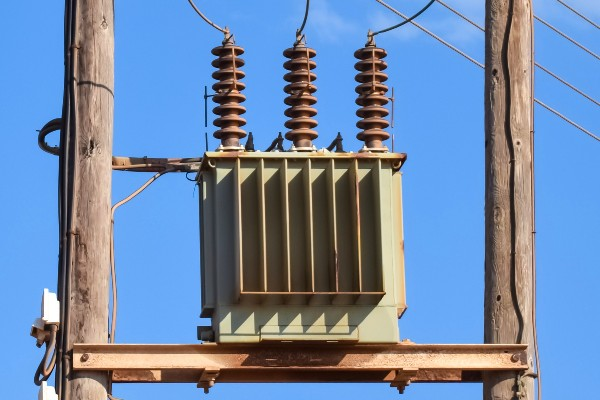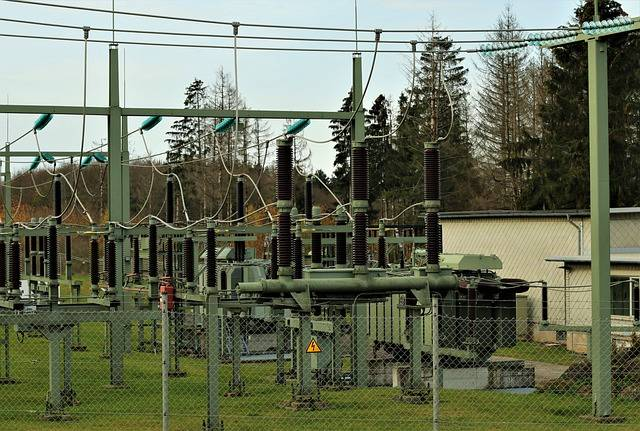Select Page

# 5 Important uses of Transformer with Explanations

Compiled by Stanley Udegbunam || Dec 09, 2020#### AFRILCATE

Transformers are electrical devices that use electromagnetic induction to pass an alternating current signal from one circuit to another.

In a hurry and won’t be able to read through the complete article?

## Here are the 5 important uses of transformer..

If you want to know how transformers perform the different functions listed above, then the remaining part of this article will guide you accordingly.

## 1. Transformers are used to regulate Voltage

The main purpose of transformers is to step up or step down the level of voltage.

It steps up the level of voltage at the generation side before transmission and distribution.

In the distribution side, transformers step down the level of voltage for commercial or domestic use.

The power voltage required by various electricity users varies according to their needs.

Therefore, it’s ideal to ship high-voltage electricity from the power station and then step it down to lower voltages when it reaches its various destinations.

The energy an appliance consumes is directly proportional to its voltage usage.uses of transformer – voltage regulation

## 2. Transformer Mitigates Power Loss and increases Energy Efficiency

In a transformer, when the voltage increases, the current flowing across the line reduces.

And when the voltage reduces, the current flowing across the line increases.

This brings us to the popular question:

### How does a transformer increase voltage while decreasing the current?

Contrary to ohm’s law (V=IR), which tells us that:

voltage increases as current increases,

transformers do not follow the postulations of ohms law.

Ohms law is only applied to ohmic device like resistors.

The total power handled by the transformer must be the same on the input and output side.

This means that Pin = Pout and from Joule-Lenz Law, we know that P = IV

Deductively,

 Iin Vin= Iout Vout

Therefore, It’s logical that when the voltage increases, the current reduces so the power transmitted remains the same.

clear right?

To mitigate power loss, the transmission voltage needs to be stepped up.

This means that increasing the transmission voltage will help reduce power loss.

Again, this will lead us to another important question:

### Why do high transmission voltages reduce power loss?

Consider an electric cooker that requires a current of 20 amperes at 240 volts.

The power required for operation will be:

P = I × V,

P = 20 × 240 = 4800 Watt.

This means the electricity transmission company, have to deliver 4800 Watt at the consumer’s end to meet this load requirement.

As this power travels through conductors over long distances, it will encounter some electrical resistance, assume 0.3 ohms.

Now, Let’s analyze 2 different cases:

#### Case 1: Transmission at 240 volts:

P= 4800 watts (as calculated above)

V= 240 volts,

I = 20 amperes,

Resistance of Transmission line= 0.3 ohm

Power loss = I2R = 202  × 0.3 = 120 watt.

The transmission company has to supply an additional 120 Watt to compensate for the power loss in the transmission line, so as to deliver exactly 4800 watts at the consumer’s end.

#### Case 2: Transmission at 11,000 volts (11kV):

If we decide to use an 11kV / 230-volt transformer to deliver the power of 4800 watts, then the current transmitted becomes:

I = P / V

I = 4800 / 11,000 = 0.436 watt.

Power loss here becomes I2R = 0.4362 × 0.3 = 0. 06 Watt.

The transmission company has to supply an additional 0.06 Watt to compensate for the power loss in the transmission line.

By simply raising the transmission voltage from 240 volts to 11000 volts, we are saving a net power loss of 120 – 0.06 = 119.94 watts.

Now, consider a real case scenario where a large amount of power (in megawatts) are being transmitted on a daily basis.

Without transformers, there will be a lot of power losses.

Therefore, transformers mitigates power loss by increasing transmission voltages which will cause a corresponding decrease in the transmission current.uses of transformer – mitigating power loss

## 3. Transformer Prevents DC from Passing from One Circuit to Another

Transformers only work with alternating current (A.C).

The device prevents direct current from passing the circuit.

### Why do transformers only work with AC?

Transformers function only with alternating current because they create a changing magnetic field which will, in turn, induce a changing voltage in a coil.

This changing voltage makes it possible for current to be transferred across ends through the principle of electromagnetic induction.

Direct current, on the other hand, is steady, they don’t cause any change in magnetic fields.

Therefore, they will produce zero induced voltage.

## 4. Transformer is used for Impedance Matching

Among the various uses of transformer, impedance matching seems to be the least talked about because of the technicality of the term.

Impedance is a measure of the opposition to current flow within a circuit or electrical component.

It encompasses the resistance and opposition caused by the physical properties of components.

The maximum power transfer theorem states that the impedance of each component must be equal in magnitude in order for the greatest amount of power to be transferred.

Matching load impedance is critical to the functionality of the circuit, especially for maximum power transfer.

The simplest way to match load impedance in AC circuits is to use a transformer.

Impedance matching transformers is useful for the following reasons:

• It helps tackle various issues caused by electrical impedance.
• They have broadband which makes it possible to work with a wide range of frequencies.
• It ensures efficient operations.uses of transformer – impedance matching

## 5. They act as an Electrical Safety Device by Stopping the Flow of Electric Current

This is another important use of transformers in everyday life.

Transformers are commonly present in circuit breakers, where they utilize a switch to automatically interrupt the flow of electricity.

By so doing, they help to prevent damage that can occur as a result of high voltage.

Such transformers are called isolation transformer and have a 1:1 turn’s ratio.

Isolation transformers don’t step voltage up or down. Instead, they serve as safety devices.